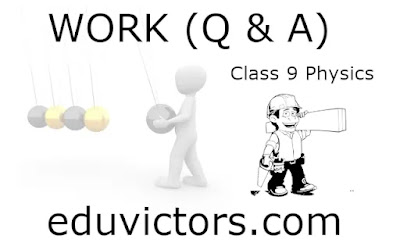CBSE Class 9 - Physics - Work (Questions and Answers)

Q1: 2 Newton-metre is how many joules?

Answer: ∵ 1 Nm = 1J ∴ 2Nm = 2J

Q2: In the CGS system, what is the unit for Work?

Q3: Is work scalar or vector?

Answer: Work is a scalar quantity (but you can have positive and negative work).

Q4: A boy pushes a book by applying a force of 10N. If the book displaces by 25cm, how much work is done by the force?

s = 25 cm = 0.25m

W = F × s

W = 10 × 0.25 = 2.5 J

Q5: A person pushes the wall by applying a force of 20N. The wall does not move. Does the person do any work? State reasons.

Answer: Since W = F × s

Here displacement s = 0.

∴ W = 0 J

Q6: A ball of mass 2kg is thrown upwards. It goes up to the height of 3m. How much work is done by the force of gravity? Is the work done positive or negative? Take g  9.8m/s².

Given,

F = mg = 2 × 9.8 = 19.6N

Displacement s = height covered = 3m

Since the direction of displacement is opposite to gravity force, negative work is done.

W = -F × s

W = -19.6 × 3 = -58.8J

It means 58.8J of work is done against gravity.

Q7(HOTS): What is the formula of Work done if force (F) acts obliquely making angle θ with the displacement s?

Q8(HOTS): A girl pushes a toy with a force of 5N obliquely at an angle of 30°. The toy moves forward by a 1.5m distance. How much work is done by the force? (cos30° = 0.5)

Given,

F = 5N

s = 1.5m

θ = 30°

W = F × s × cosθ

= 5 × 1.5 × cos30°

= 5 × 1.5 × 0.5

= 3.75 J

Q9: A horse pulls the cart. Both the horse and the cart move forward. Is the work done by the horse positive or negative?

Answer: Positive work since the applied force (by the horse) and the displacement are in the same direction.

Q10: A boy moves a stone in a circle by attaching it to a string. If the tension of the string is 3N and the length of the string is 0.5 then how much work is done by the string?

Since the tension (centripetal force) is perpendicular to the displacement, zero work is done.

Q11(HOTS): If a Force-displacement graph is plotted by taking displacement on the x-axis and the Force on the y-axis, the area under Force-displacement represents what?

Q12(HOTS): Identify the work done is positive or negative in the following F-s graph for areas labelled as 'A' and 'B'.

Area A = +ve work

Area B = -ve work1.